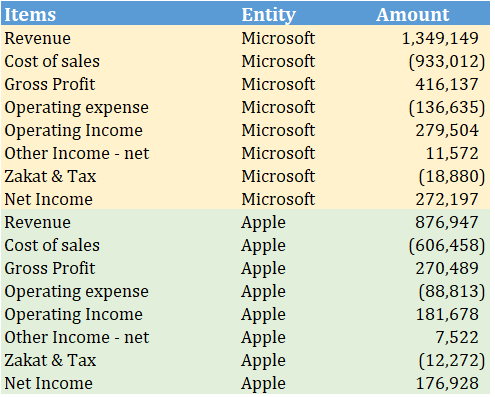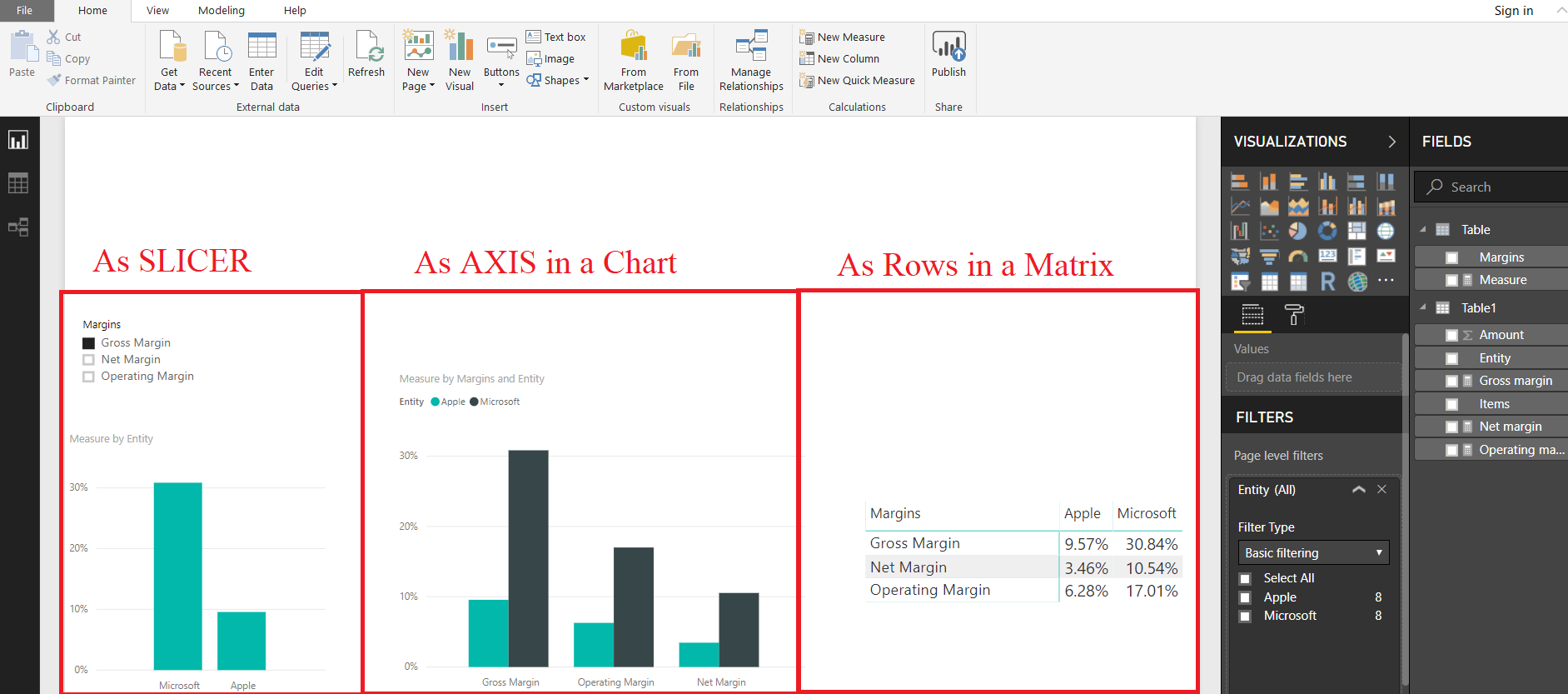# Use MEASURES in Slicers, Rows and Chart Axis

So we have this sample financial data of 2 technology giants..Microsoft and AppleUsing this Data we create 3 MEASURES to compute Profitability Ratios of these companies

```Gross margin =
DIVIDE (
CALCULATE ( SUM ( Table1[Amount] ), Table1[Items] = "Gross Profit" ),
CALCULATE ( SUM ( Table1[Amount] ), Table1[Items] = "Revenue" )
)

Operating margin =
DIVIDE (
CALCULATE ( SUM ( Table1[Amount] ), Table1[Items] = "Operating Income" ),
CALCULATE ( SUM ( Table1[Amount] ), Table1[Items] = "Revenue" )
)

Net margin =
DIVIDE (
CALCULATE ( SUM ( Table1[Amount] ), Table1[Items] = "Net Income" ),
CALCULATE ( SUM ( Table1[Amount] ), Table1[Items] = "Revenue" )
)```

You can put these MEASURES in “VALUES” section for any visual but

You can NOT put MEASURE in any other section like Slicers, Axis in a Chart or Rows of Matrix etc.

Only a column (including Calculated column) can be placed in Slicers, Axis in a Chart or Rows of Matrix etc.But there is a trick to use MEASURES in slicers, Chart Axis or Matrix Rows etc

The trick is to create a separate table with a column containing the names of the MEASURES…and then create a formula to link MEASURES to these Column Values

## FIRST STEP

Create a calculated table from the Modelling Tab to get MEASURE names in a column. (Alternatively you can manually create a Table by inputting information from Home Tab>>Enter Data)

```Table =
DATATABLE (
"Margins", STRING,
{
{ "Gross Margin" },
{ "Operating Margin" },
{ "Net Margin" }
}
)```

## STEP 2

Now we can create a MEASURE to link the Column Values to original profitability MEASURES

```Measure =
VAR myvalue =
SELECTEDVALUE ( 'Table'[Margins] )
RETURN
SWITCH (
TRUE (),
myvalue = "Gross Margin", [Gross margin],
myvalue = "Operating Margin", [Operating margin],
myvalue = "Net Margin", [Net margin]
)```

## STEP 3

Now you can use the combination of STEP 1 (Column created) and STEP 2 (MEASURE) to get the original MEASURES in SLICERS, Chart Axis, or Matrix Rows etc